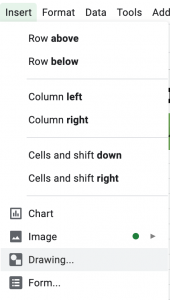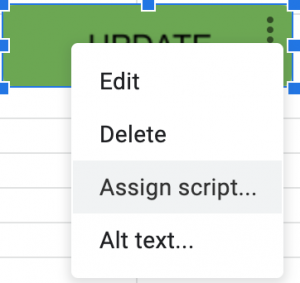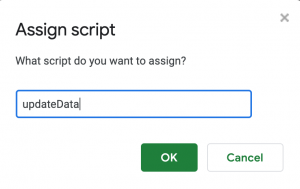# How To Update A Range Of Formulas in Google Scripts

May 6, 2019In this blog post, I’ll show you how to update a range of cells in a Google Sheet that have formulas. This is useful if you want to create an update button that triggers the refresh of information in cells that pull in data from an API.

## The Script

The script has several cool features:

• Ability to assign default ranges
• Ability to select a range
• Ability to manually define a range
• Manually set sheets to update or update all sheets
```/**
* Get all sheet names from active spreadsheet
*/
function sheetNames() {
var sheetNames = [];
for (var i = 0; i < sheets.length; i++) sheetNames.push( sheets[i].getName() )
return sheetNames;
}

/**
* Update all formula data in cell range
*/
function updateData() {
activeSheetName = activeSheet.getSheetName(),
activeRange     = activeSheet.getActiveRange(),
sheets          = sheetNames() // OR define an array of sheet names: ['Sheet1', 'Sheet2'],
cellRange       = (activeRange.getValues().length > 1) ? activeRange.getA1Notation() : Browser.inputBox('Enter your range'),
range           = '';

// loop through all sheets
sheets.forEach(function(sheet) {
var originalFormulas = [],
range            = activeRange,
defaultRanges    = {
'Sheet1': 'I2:I64',
'Sheet2': 'C2:C10'
},

// set cell range if entered, ortherwise fall back to default
cellRange = cellRange ? cellRange : defaultRanges[sheet];

// set range to active range, otherwise fall back to defined cell range

originalFormulas = range.getFormulas();

// modify formulas
const modified = originalFormulas.map(function (row) {
return row.map(function (col) {
return col.replace('=', '?');
});
});

// update formulas
range.setFormulas(modified);

// return formulas to original
range.setFormulas(originalFormulas);
});
}```

Breaking this down, we can see a few things going on.

• Line 19: You can either loop through all sheets or define an array of sheet names to loop through
• Lines 27-30: You can define specific default ranges for particular sheets

The main trick to this function is that we are updating the formula on line 43. There is no “update” function in Google Scripts, so we have to trick the script to work in the way we want it to. In this case, we are replacing the = with a ? and then switching back to the original formula.

## Connecting The Script To A ButtonStep 1Step 2Step 3